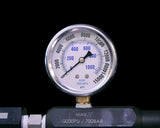# How to Find the Optimal Pressure for Your Rosin Press

Choosing the best pressure for a rosin press can be a bit tricky. The key thing to remember? It's all about Platen PSI (pounds per square inch). We'll explain what that means and help you figure out the right amount.

## How Much Pressure Do I Need?

The optimal pressure depends on what you're pressing. Based on what most folks in the industry say, here are the recommended Platen PSI levels for pressing rosin, depending on your starting material:

• Flower Rosin: Between 1,200 and 2,500 Platen PSI (more pressure)
• Hash Rosin: Between 700 and 2,000 Platen PSI (less pressure)

If you go above these ranges and apply too much pressure, you might tear parchment paper, rosin bags, etc. If you go below, you will not get as much yield and might need higher temperatures (less terpenes).

## Figuring Out Your Platen PSI

Platen PSI is just a fancy way to talk about the pressure you're putting on your material. It's like squeezing a sponge—you want to get all the water out without tearing the sponge. Here's a simple way to calculate Platen PSI:

1. Measure the size of your rosin bag (not the size of the heat plate). Let's say it's 3"x6"—that's 18 square inches.
2. Check the force you're using. For example, if you're using half the strength of a 20-ton rosin press, that's 10 tons or 20,000 lbs of force.
3. Now, divide the force by the bag's size. For our example: 20,000 lbs ÷ 18 square inches = 1,111 Platen PSI.

## Making Sense of Pressure GaugesThose numbers on the pressure gauges of hydraulic rosin presses? They're telling you about the internal hydraulic pressure, not what the heat plates are actually applying to your material.

On a Lowtemp V2 Rosin Press, 10K psi means the full force of the 20 ton cylinder. This means every 1,000 psi on the pressure gauge comes out to 2 tons or 4,000 lbs of total force.

## Never Do Math AgainWe created a solution in our LT3 Heat Controller! This system is fully backwards compatible with all of our rosin presses.

Simply input the size of your bag and the controller will automatically calculate pressure in Platen PSI via an inline pressure sensor.

It will also transmit this data to a local device where you can map pressure, time, and temperature all in one location.

## Get Great Results Every Time

Choosing the right rosin press pressure doesn't have to be rocket science. Remember the recommended Platen PSI levels, do a bit of simple math, and you'll get great results every time. And whenever you’re in doubt about the pressure required, always refer to your press's guidelines or ask an expert.